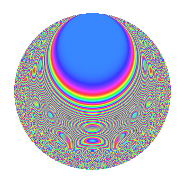Properties

 Label 6019.2.a.dLevel 6019 Weight 2 Character orbit 6019.a Self dual Yes Analytic conductor 48.062 Analytic rank 0 Dimension 123 CM No

Related objects

Newspace parameters

 Level: $$N$$ = $$6019 = 13 \cdot 463$$ Weight: $$k$$ = $$2$$ Character orbit: $$[\chi]$$ = 6019.a (trivial)

Newform invariants

 Self dual: Yes Analytic conductor: $$48.0619569766$$ Analytic rank: $$0$$ Dimension: $$123$$ Fricke sign: $$-1$$ Sato-Tate group: $\mathrm{SU}(2)$

$q$-expansion

The dimension is sufficiently large that we do not compute an algebraic $$q$$-expansion, but we have computed the trace expansion.

 $$\operatorname{Tr}(f)(q) =$$ $$123q$$ $$\mathstrut +\mathstrut 10q^{2}$$ $$\mathstrut +\mathstrut q^{3}$$ $$\mathstrut +\mathstrut 136q^{4}$$ $$\mathstrut +\mathstrut 46q^{5}$$ $$\mathstrut +\mathstrut 16q^{6}$$ $$\mathstrut +\mathstrut 12q^{7}$$ $$\mathstrut +\mathstrut 30q^{8}$$ $$\mathstrut +\mathstrut 154q^{9}$$ $$\mathstrut +\mathstrut O(q^{10})$$ $$\operatorname{Tr}(f)(q) =$$ $$123q$$ $$\mathstrut +\mathstrut 10q^{2}$$ $$\mathstrut +\mathstrut q^{3}$$ $$\mathstrut +\mathstrut 136q^{4}$$ $$\mathstrut +\mathstrut 46q^{5}$$ $$\mathstrut +\mathstrut 16q^{6}$$ $$\mathstrut +\mathstrut 12q^{7}$$ $$\mathstrut +\mathstrut 30q^{8}$$ $$\mathstrut +\mathstrut 154q^{9}$$ $$\mathstrut +\mathstrut 5q^{10}$$ $$\mathstrut +\mathstrut 53q^{11}$$ $$\mathstrut -\mathstrut 6q^{12}$$ $$\mathstrut -\mathstrut 123q^{13}$$ $$\mathstrut +\mathstrut 21q^{14}$$ $$\mathstrut +\mathstrut 29q^{15}$$ $$\mathstrut +\mathstrut 166q^{16}$$ $$\mathstrut -\mathstrut 35q^{17}$$ $$\mathstrut +\mathstrut 28q^{18}$$ $$\mathstrut +\mathstrut 23q^{19}$$ $$\mathstrut +\mathstrut 93q^{20}$$ $$\mathstrut +\mathstrut 72q^{21}$$ $$\mathstrut +\mathstrut 8q^{22}$$ $$\mathstrut +\mathstrut 42q^{23}$$ $$\mathstrut +\mathstrut 55q^{24}$$ $$\mathstrut +\mathstrut 153q^{25}$$ $$\mathstrut -\mathstrut 10q^{26}$$ $$\mathstrut +\mathstrut 7q^{27}$$ $$\mathstrut +\mathstrut 39q^{28}$$ $$\mathstrut +\mathstrut 86q^{29}$$ $$\mathstrut +\mathstrut 44q^{30}$$ $$\mathstrut +\mathstrut 16q^{31}$$ $$\mathstrut +\mathstrut 70q^{32}$$ $$\mathstrut +\mathstrut 40q^{33}$$ $$\mathstrut +\mathstrut 10q^{34}$$ $$\mathstrut +\mathstrut 6q^{35}$$ $$\mathstrut +\mathstrut 222q^{36}$$ $$\mathstrut +\mathstrut 52q^{37}$$ $$\mathstrut +\mathstrut 12q^{38}$$ $$\mathstrut -\mathstrut q^{39}$$ $$\mathstrut +\mathstrut 14q^{40}$$ $$\mathstrut +\mathstrut 80q^{41}$$ $$\mathstrut +\mathstrut 29q^{42}$$ $$\mathstrut +\mathstrut 2q^{43}$$ $$\mathstrut +\mathstrut 143q^{44}$$ $$\mathstrut +\mathstrut 137q^{45}$$ $$\mathstrut +\mathstrut 39q^{46}$$ $$\mathstrut +\mathstrut 45q^{47}$$ $$\mathstrut -\mathstrut 27q^{48}$$ $$\mathstrut +\mathstrut 163q^{49}$$ $$\mathstrut +\mathstrut 102q^{50}$$ $$\mathstrut +\mathstrut 48q^{51}$$ $$\mathstrut -\mathstrut 136q^{52}$$ $$\mathstrut +\mathstrut 117q^{53}$$ $$\mathstrut +\mathstrut 75q^{54}$$ $$\mathstrut +\mathstrut 20q^{55}$$ $$\mathstrut +\mathstrut 88q^{56}$$ $$\mathstrut +\mathstrut 67q^{57}$$ $$\mathstrut +\mathstrut 56q^{58}$$ $$\mathstrut +\mathstrut 88q^{59}$$ $$\mathstrut +\mathstrut 96q^{60}$$ $$\mathstrut +\mathstrut 57q^{61}$$ $$\mathstrut -\mathstrut 13q^{62}$$ $$\mathstrut +\mathstrut 48q^{63}$$ $$\mathstrut +\mathstrut 228q^{64}$$ $$\mathstrut -\mathstrut 46q^{65}$$ $$\mathstrut +\mathstrut 28q^{66}$$ $$\mathstrut +\mathstrut 43q^{67}$$ $$\mathstrut -\mathstrut 56q^{68}$$ $$\mathstrut +\mathstrut 92q^{69}$$ $$\mathstrut +\mathstrut 14q^{70}$$ $$\mathstrut +\mathstrut 90q^{71}$$ $$\mathstrut +\mathstrut 98q^{72}$$ $$\mathstrut +\mathstrut 25q^{73}$$ $$\mathstrut +\mathstrut 80q^{74}$$ $$\mathstrut +\mathstrut 21q^{75}$$ $$\mathstrut +\mathstrut 75q^{76}$$ $$\mathstrut +\mathstrut 112q^{77}$$ $$\mathstrut -\mathstrut 16q^{78}$$ $$\mathstrut +\mathstrut 36q^{79}$$ $$\mathstrut +\mathstrut 208q^{80}$$ $$\mathstrut +\mathstrut 231q^{81}$$ $$\mathstrut -\mathstrut 27q^{82}$$ $$\mathstrut +\mathstrut 93q^{83}$$ $$\mathstrut +\mathstrut 175q^{84}$$ $$\mathstrut +\mathstrut 77q^{85}$$ $$\mathstrut +\mathstrut 199q^{86}$$ $$\mathstrut +\mathstrut 15q^{87}$$ $$\mathstrut +\mathstrut 43q^{88}$$ $$\mathstrut +\mathstrut 140q^{89}$$ $$\mathstrut +\mathstrut 11q^{90}$$ $$\mathstrut -\mathstrut 12q^{91}$$ $$\mathstrut +\mathstrut 93q^{92}$$ $$\mathstrut +\mathstrut 140q^{93}$$ $$\mathstrut +\mathstrut 4q^{94}$$ $$\mathstrut +\mathstrut 23q^{95}$$ $$\mathstrut +\mathstrut 105q^{96}$$ $$\mathstrut +\mathstrut 43q^{97}$$ $$\mathstrut +\mathstrut 67q^{98}$$ $$\mathstrut +\mathstrut 140q^{99}$$ $$\mathstrut +\mathstrut O(q^{100})$$

Embeddings

For each embedding $$\iota_m$$ of the coefficient field, the values $$\iota_m(a_n)$$ are shown below.

For more information on an embedded modular form you can click on its label.

Label $$a_{2}$$ $$a_{3}$$ $$a_{4}$$ $$a_{5}$$ $$a_{6}$$ $$a_{7}$$ $$a_{8}$$ $$a_{9}$$ $$a_{10}$$
1.1 −2.75439 −0.861643 5.58668 3.86069 2.37330 2.91861 −9.87912 −2.25757 −10.6339
1.2 −2.74057 −2.54852 5.51070 −0.432732 6.98439 3.36424 −9.62130 3.49496 1.18593
1.3 −2.71192 −2.81974 5.35451 4.11778 7.64691 −3.85646 −9.09715 4.95095 −11.1671
1.4 −2.69112 1.73469 5.24211 2.53592 −4.66825 −4.16450 −8.72491 0.00913932 −6.82446
1.5 −2.68053 2.57455 5.18522 0.534092 −6.90114 −0.399106 −8.53805 3.62830 −1.43165
1.6 −2.65517 −0.380089 5.04992 1.30385 1.00920 0.922271 −8.09806 −2.85553 −3.46193
1.7 −2.60439 −3.42443 4.78284 −1.92258 8.91854 −3.26289 −7.24759 8.72671 5.00714
1.8 −2.52129 0.498790 4.35689 −0.537697 −1.25759 −2.34122 −5.94240 −2.75121 1.35569
1.9 −2.52093 1.18363 4.35509 −0.839879 −2.98385 2.35424 −5.93701 −1.59902 2.11728
1.10 −2.49040 −2.13188 4.20211 −1.49942 5.30925 −0.951507 −5.48415 1.54493 3.73417
1.11 −2.47080 2.36731 4.10488 −1.23232 −5.84916 4.18566 −5.20074 2.60415 3.04483
1.12 −2.26767 −1.90418 3.14232 −3.16055 4.31804 −3.88251 −2.59040 0.625886 7.16707
1.13 −2.26442 2.63903 3.12758 3.87429 −5.97587 0.802453 −2.55330 3.96450 −8.77301
1.14 −2.25898 −0.131578 3.10301 3.21477 0.297232 0.192917 −2.49169 −2.98269 −7.26212
1.15 −2.22237 3.41646 2.93895 1.79804 −7.59266 3.28634 −2.08670 8.67221 −3.99592
1.16 −2.19076 0.906147 2.79943 −2.56920 −1.98515 −1.27799 −1.75137 −2.17890 5.62849
1.17 −2.17075 −1.42264 2.71217 −2.44924 3.08820 1.96298 −1.54596 −0.976102 5.31670
1.18 −2.16481 1.72504 2.68638 −0.175994 −3.73438 −2.81780 −1.48588 −0.0242310 0.380992
1.19 −2.14591 −1.26575 2.60492 3.62169 2.71619 0.00950737 −1.29811 −1.39787 −7.77182
1.20 −2.11210 −0.313775 2.46095 −4.01398 0.662722 2.82593 −0.973578 −2.90155 8.47792
See next 80 embeddings (of 123 total)
 $$n$$: e.g. 2-40 or 990-1000 Embeddings: e.g. 1-3 or 1.123 Significant digits: Format: Complex embeddings Normalized embeddings Satake parameters Satake angles

Inner twists

This newform does not have CM; other inner twists have not been computed.

Atkin-Lehner signs

$$p$$ Sign
$$13$$ $$1$$
$$463$$ $$-1$$

Hecke kernels

This newform can be constructed as the kernel of the linear operator $$T_{2}^{123} - \cdots$$ acting on $$S_{2}^{\mathrm{new}}(\Gamma_0(6019))$$.